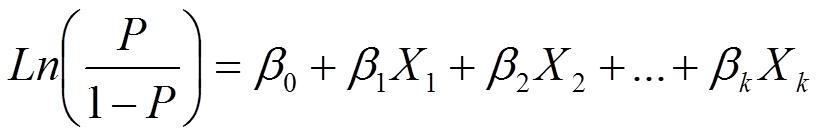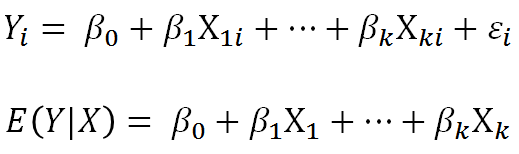# Link Functions and Errors in Logistic Regression

I recently held a free webinar in our The Craft of Statistical Analysis program about Binary, Ordinal, and Nominal Logistic Regression.

It was a record crowd and we didn’t get through everyone’s questions, so I’m answering some here on the site. They’re grouped by topic, and you will probably get more out of it if you watch the webinar recording. It’s free.

The following questions refer to this logistic regression model:#### Q: So the link function is everything on the left of the equals sign?

Yes, exactly. The right side looks pretty much like every other regression equation you’ve seen.

But the left side has a link function instead of Y. Since P is  the conditional mean of Y, this ugly mess is simply a function of the mean. That’s the definition of a link function — a function of the mean of Y.

And although it looks ugly at first, it’s really not so bad once you learn more about logistic regression. It’s simply the natural log of the odds.

#### Q: What is the base of the log? e or 10 or maybe something else?

It’s e.

This is the natural log.

#### Q: Why isn’t there an error term in the logit model?

It’s because we’re only modeling the mean here, not each individual value of Y.

Logistic Regression is one type of Generalized Linear Model and they all have that same feature. Rather than model each value of Y with the predicted mean plus an error term, it simply models the predicted mean.

This is related to the two ways we can write a linear model. (See the next question, below). For generalized linear models, we can only use the second form AND we have to apply a link function to that predicted mean on the left.

#### Q:  Is there an error term in that form of the linear model also?

(This question refers to these two ways of writing the linear regression model, which we reviewed at the beginning of the webinar):Only the first has an error term, because here we are writing a model for each of the i individual values of Y. You’ll notice that not only does the error term have a subscript i, but so do all the Xs and Y.

The second one doesn’t need an error term, because the left side of the equation is not each value of Y, but the mean of Y at given values of X. That’s the value on the regression line.

We needed the error term in the first equation to move us up or down from the regression line to get to the actual data point. Here we are just staying on the regression line.

That’s what we do in generalized linear models, like logistic regression. Just model the regression line, but we are unable at all to model individual points.Binary, Ordinal, and Multinomial Logistic Regression for Categorical Outcomes
Get beyond the frustration of learning odds ratios, logit link functions, and proportional odds assumptions on your own. See the incredible usefulness of logistic regression and categorical data analysis in this one-hour training.

1.Himayatullah Khan says

Hi Karen Grace-Martin
Thanks for being there to reply to our queries. I would appreciate your guidance regarding the logit and probit regressions. In classical linear regression models, we apply some tests to test assumptions like heteroskedasticity, autocorrelation, normality, multicollinearity, etc. Do we need to test also some assumptions in logit and probit regression models like in OLS?
Himmy

•Karen Grace-Martin says

Yes, every model and test has assumptions. They are, of course, different for logistic and probit than they are for linear models.

2.Prafulla Kumar Nath says

Is there an error term in that form of the linear model also?
I ave a query about the above equation, if you can shed some light over it.

If error term is optional ( where we may put / may not put a subscript i, then I will prefer not to put. It is because with error term question about endogeneity will come. By not putting error can we claim in logistic equation endogeneity will not occur?

•Karen Grace-Martin says

It’s not that an error term is optional in linear regression. What’s optional in linear regression is whether you write the model about the actual values of Y or the expected value of Y. If it’s the actual values of Y, you MUST include an error term. If it’s the expected values of Y (Y-hat), then you MUST NOT include an error term.

Logistic and other generalized linear models can only do the latter.

3.unekwu onuche says

Thank you for this eye-opening presentation.

4.Axf says

Thanks , that’s a really useful post. I have a question – does this mean that glm are not useful for predictive modelling? I want to predict some data that looks like it fits a gamma distribution, so I thought I’d use a glm with gamma link. But if “we are unable at all to model individual points”, does that mean glm is of no use here? How can I add an error to the predicted mean?

•Karen Grace-Martin says

HI Axf,

No, it doesn’t. In any regression model, the predictions are always about the conditional mean, not the individual points. The errors are there, but considered a nuisance term, not what we’re interested in.

5.Geraud N. says

Nice presentation. But is there any endogeneity in logistic regression? if yes how to address it?

•Karen Grace-Martin says

Hi Geraud,

Endogeneity is about the inference, not about the model. So yes, it’s possible in logistic regression. In my experience, this is an issue only for econometricians due to the types of inferences they’re trying to make based on the types of data collection methods, so I’m not sure how they address it. 🙂

6.JUAN CARLOS SALAZAR URIBE says

Thanks Dr. Karen Grace-Martin, your post has been very very helpful for me. have a nice day

Please note that, due to the large number of comments submitted, any questions on problems related to a personal study/project will not be answered. We suggest joining Statistically Speaking, where you have access to a private forum and more resources 24/7.